# Concave And Convex Lenses: Image Formation

Spherical lenses are the lenses formed by bounding two spherical transparent surfaces together. In general, there are two types of spherical lenses. So, lenses formed by binding two spherical surfaces bulging outward are known as convex lenses while the lenses formed by binding two spherical surfaces such that they are curved inward are known as concave lenses. This is one of the basic difference between concave and convex lenses.

Convex lenses are also known as converging lenses since the rays converge after falling on the convex lens while the concave lenses are known as diverging lenses as the rays diverge after falling on the concave lens. In this article, we will learn about image formation by concave and convex lenses.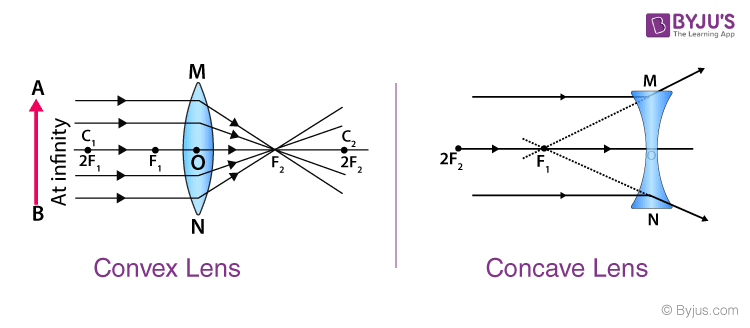## Guidelines for rays falling on the concave and convex lenses

1. When a ray strikes concave or convex lenses obliquely at its pole, it continues to follow its path.
2. When a ray, parallel to the principal axis strikes concave or convex lenses, the reflected ray passes through a focus on the principal axis.
3. When a ray, passing through focus strikes concave or convex lenses, the reflected ray will pass parallel to the principal axis.

## Image Formation by Concave and Convex Lenses:

### Convex Lenses

• When an object is placed at infinity, the real image is formed at the focus. The size of the image is much smaller than that of the object.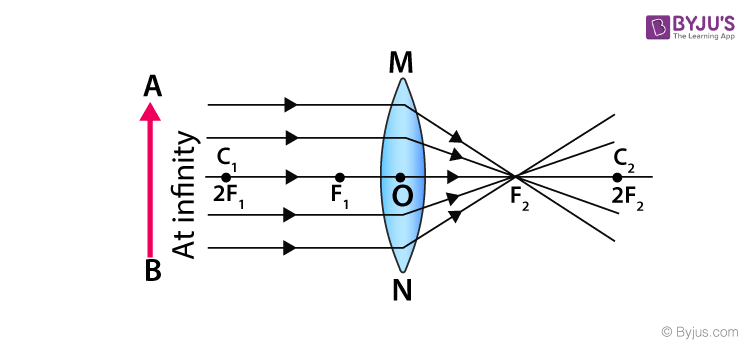• When an object is placed behind the center of curvature, the real image is formed between the center of curvature and focus. The size of the image is the same as compared to that of the object.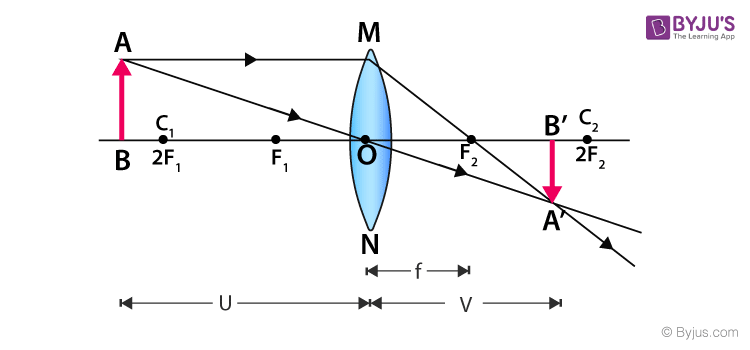• When an object is at the center of curvature, the real image is formed at the other center of curvature. The size of the image is the same as compared to that of the object.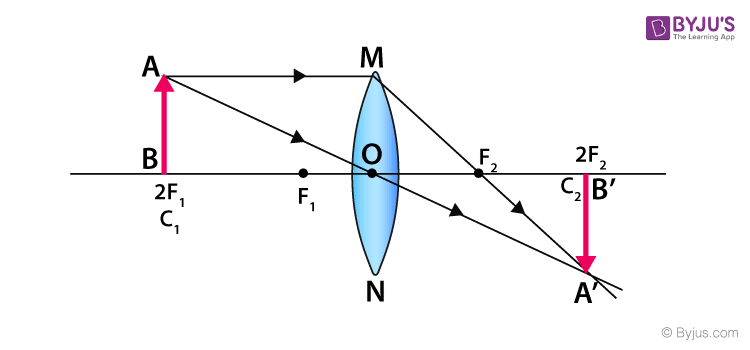• When an object is placed in between the center of curvature and focus, the real image is formed behind the center of curvature. The size of the image is smaller than that of the object.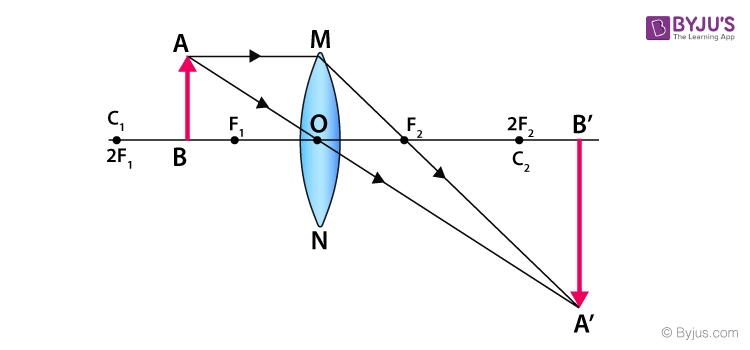• When an object is placed at the focus, a real image is formed at infinity. The size of the image is much larger than that of the object.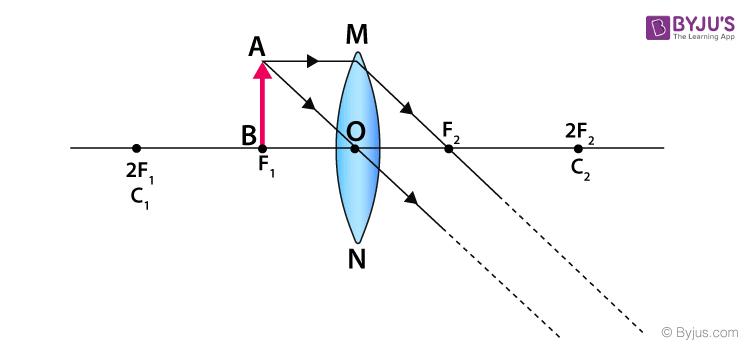• When an object is placed in between focus and pole, a virtual image is formed. The size of the image is larger than that of the object.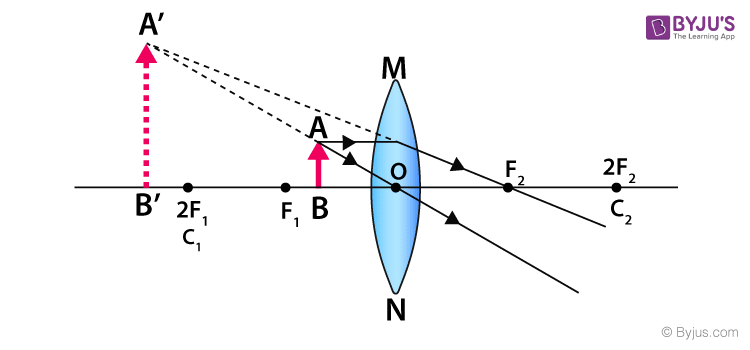### Concave Lenses

• When an object is placed at infinity, a virtual image is formed at the focus. The size of the image is much smaller than that of the object.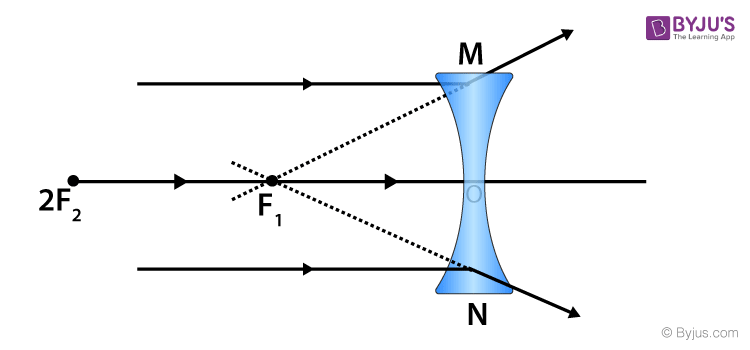• When an object is placed at a finite distance from the lens, a virtual image is formed between the pole and the focus of the convex lens. The size of the image is smaller than that of the object.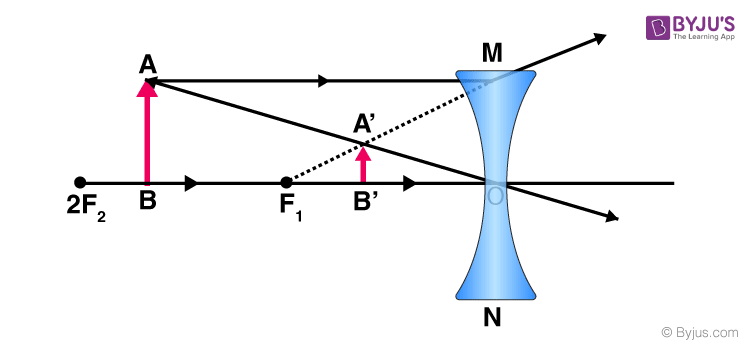## Summary of Image Formation by Concave and Convex Lens

 Image formation by Concave Lens Object Location Image Location Image Nature Image Size Infinity At F2 Virtual and Erect Highly Diminished Beyond Infinity and Zero Between F1 and Optical center Virtual and Erect Diminished
 Image formation by Convex Lens Object location Image location Image nature Image size Infinity At F2 Real and Inverted Diminished Beyond 2 F1 Between 2F2 and F2 Real and Inverted Diminished Between 2F1 and F1 Beyond 2F2 Real and Inverted Enlarged At F1 At infinity Real and Inverted Enlarged At 2 F1 At 2F2 Real and Inverted Same size Between F1 and 0 On the same side as the object Virtual and Erect Enlarged

Stay tuned with BYJU’S to learn more about image formation by concave and convex lenses and explore more on physics formulas and calculators.

#### 1 Comment

1. Banu Teja

I need this very much